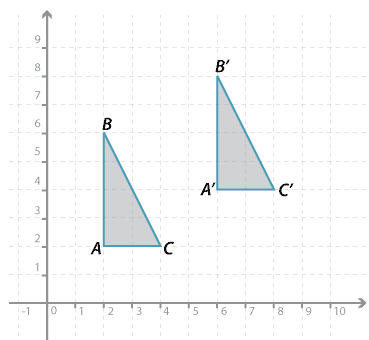## Content description

Describe translations, reflections in an axis, and rotations of multiples of 90 ° on the Cartesian plane using coordinates. Identify line and rotational symmetries (ACMMG181)

Source: Australian Curriculum, Assessment and Reporting Authority (ACARA)

### Translations on the Cartesian plane using coordinatesDetailed description

When a figure is shifted in the plane without being turned it is called a translation. To describe a translation it is enough to say how far left or right and how far up or down the figure has moved.

The $$\triangle ABC$$ in the diagram has been translated to a new position on the Cartesian plane, and the new triangle has been labelled $$\triangle A^\prime B^\prime C^\prime$$. You can see that it has shifted 4 units right and 2 units up. The point A has moved to $$A^\prime$$ and $$B$$ to $$B^\prime$$ and $$C$$ to $$C^\prime$$.

The image of a point is always written with a dash attached. Thus the image of $$A$$ is written $$A^\prime$$ (read '$$A$$ prime' or '$$A$$ dash'). The image of $$\triangle ABC$$ is written $$\triangle A^\prime B^\prime C^\prime$$.

The translation has not changed the side lengths or the angles of the triangle. We see:

$$A(2, 2) \rightarrow A^\prime (6, 4);$$ $$B(2, 6) \rightarrow B^\prime (6, 8); C(4, 2) \rightarrow C^\prime (8, 4).$$

In general, $$P(x, y) \rightarrow P^\prime (x + 4, y + 2).$$